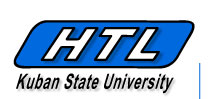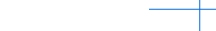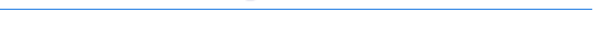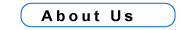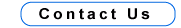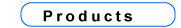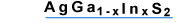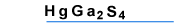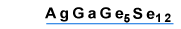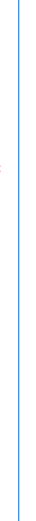AgGaGe5Se12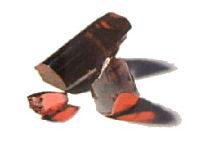Structural and Physical Properties
 Crystal symmetry and class Orthorhombic, C2v ( mm2 ) Space group C192v Cell parameters(A) a = 12.320, b = 23.64, c = 7.255 Melting point (oC) 713 ± 10 Optical homogeneity Dn < 1*10-4 Density (gram/cm3) 4.78 Absorption coefficient (cm -1) < 0.005Optical and NLO PropertiesOptical transmission ( mm ) 0.594 to 16.45 Fundamental absorption edge ( mm ) 0.56 Energy gap (eV) 2.2 Surface damage threshold ( t = 10 ns, l = 1.06 mm) - 50 MW/cm2Dispersion of refractive indices(Experimental values)l (mm) nx ny nz 1.064 2.4215 2.5683 2.5803
Nonlinear optical coefficients ( pm/V ):d31 = ± 28; d32 = ± 14.6; d33 = ± 14.6. X - c, Y - a, Z - b; At l = 1.064 mm 2V = 149.5o
dij defined in the abc frame (mm2 point group, c - polar axis)

Dispersion of refractive indices ( Sellmeier equations, l in mm )n2x = 5.7182737 - 24.729565 / ( 250.0 - l2 ) - 0.24365570 / ( 0.12550405 - l2 );
n2y = 6.5626531 - 116.75498 / ( 400.0 - l2 ) - 0.32805600 / ( 0.12928073 - l2 );
n2z = 6.6688973 - 136.03294 / ( 400.0 - l2 ) - 0.33109498 / ( 0.12679454 - l2 ).

Types of possible nonlinear processes in the principal planes
of the AgGaGe5Se12 and effective nonlinearity deff
 XY plane YZ plane XZ plane q < V oe - e, eo - e, deff = 0 oe - o, eo - o, deff = 0 oo - e, deff = d31 cosq; oo - e, deff = d32 sinj ee - o, deff = d32 sin2q + d31 cos2q oe - e, eo - e, deff = 0

Application

The AgGaGe5Se12 crystals can be used for frerquency doubling and three-frequency mixing. Parametric oscillators and amplifiers based of AgGaGe5Se12 can generate continuously tunable radiation in the spectral range from 1.2 to 15.6 mm with suitable pump laser.